Dietrich Zawischa Contact

# Mie Scattering

The exact solution for the scattering of light by spherical particles of given refractive index and absorption coefficient has been given by Gustav Mie in 1908. The method is known as partial waves expansion. Mie deals primarily with colloidal metal solutions, with particle sizes up to 180 nm, estimating that only a small number of partial waves needs to be considered in that case.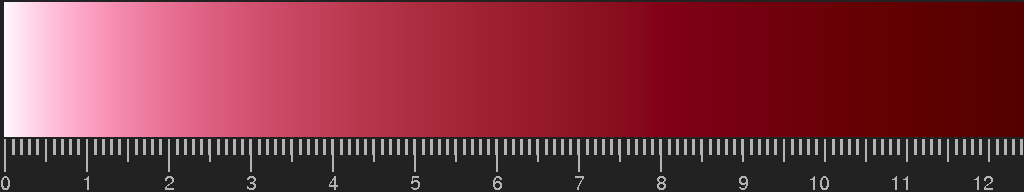Colour of gold-ruby glass, computed for spherical particles with radius 7 nm,

(Experimental data: http://refractiveindex.info/). The scale gives the thickness, multiplied by the density of the particles and the cross section area of a particle.

Mie then writes:

"In contrast to that, e.g. for the rainbow problem, which could be tackled with the method employed here, a pretty large number of partial waves would have to be accounted for and one would encounter very serious computational difficulties."
"Dagegen müßte man z.B. bei dem Regenbogenproblem, das man auch mit der hier angewandten Methode in Angriff nehmen könnte, eine ziemlich große Zahl Partialwellen berücksichtigen und würde auf sehr große rechnerische Schwierigkeiten stoßen." (p. 402)

Nonetheless, the theory has been applied to the rainbow problem. In his standard work on "Light Scattering by Small Particles", H.C. van de Hulst writes 1957: "There is now an impressive body of tabulated numerical results based on the rigorous Mie formulae. […] The bulk of the computations […], terminating in the automatic printing of all Mie coefficients, was made by the electronic ENIAC machine. The Legendre functions πn(θ) and τn(θ) were computed with an IBM punched card machine. The final multiplications and additions giving the scattering diagram, first complex amplitude, then intensity, were also made on a punched card machine."

The serious computational difficulties have been overcome by skilful programming. In 1983, Bohren and Huffmann published a FORTRAN code in their book . Today, everybody who wants can use this code on a PC and obtain results in a few seconds. There are several websites which offer applications based on the Bohren-Huffmann Mie-subroutine.

Atmospheric phenomena which can be reproduced are rainbows, fogbows, aureoles (or coronae) around the sun or moon, and the glory.

Here are examples:

#### Primary and secondary rainbow

Averaging over the sun's disc has been performed, and a grey background is assumed with luminosity L=0.4.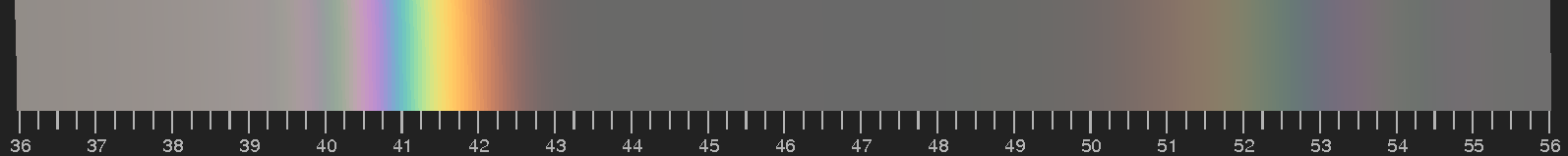Rainbow colours. Gaussian distribution of droplet diameters with dave= 0.5 mm, standard deviation Δd = 0.06 mm. The scale gives the angle in degrees, measured from the antisolar point.

#### Aureole (or corona)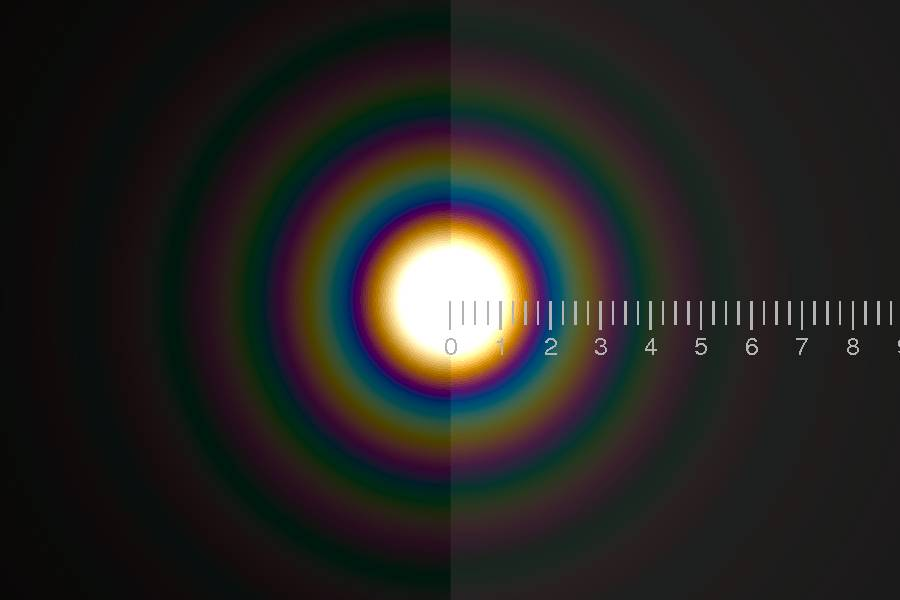Comparison of diffraction by a black circular disc (or a circular hole in a diaphragm) with radius 10 μm on the left hand side and Mie scattering by a water drop of the same radius on the right. A point light source is assumed.

Thus the aureole or corona is easily understood by diffraction, and the diffraction pattern of a circular obstacle matches the colours very well.

#### Glory

There is no simple approximation for the colours of the glory, in particular none in terms of ray optics.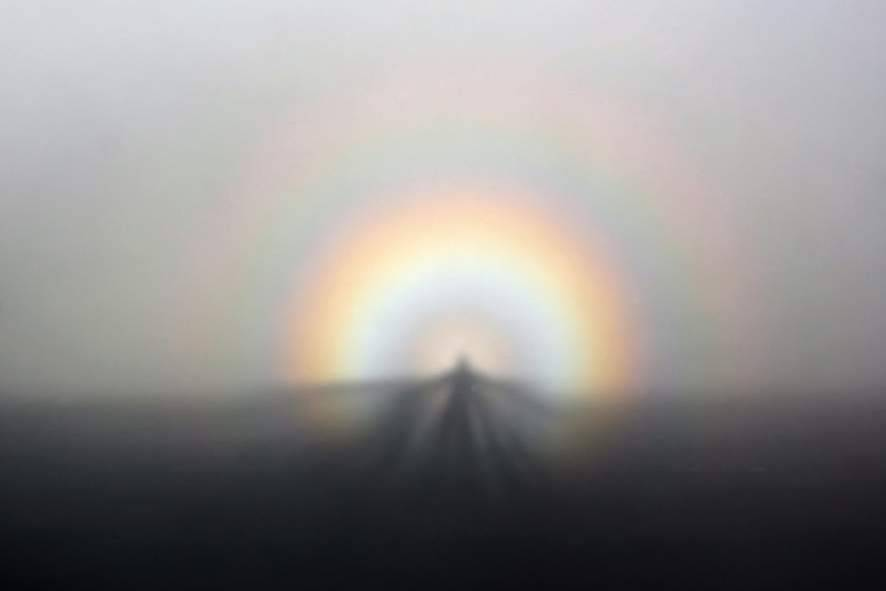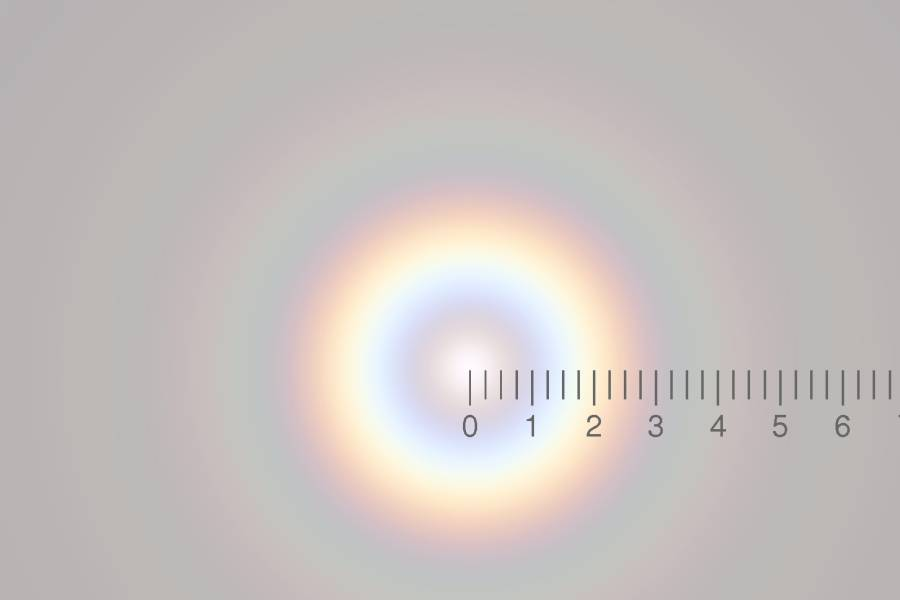Glory and Brocken spectre seen from the Golden Gate Bridge. Photo: "Brocken Inaglory", Source: Wikipedia, license CC BY-SA 3.0 Computed solar glory for average droplet radius 8 μm, Gaussian distribution of radii with standard deviation of 1.36 μm. Scale: degrees from antisolar point.

### Using the BHMIE subroutine

#### Input parameters

The subroutine BHMIE requires the input parameters

X: ratio of the circumference of the sphere and the wavelength:

X=2πa/λmedium,

where a is the radius of the sphere and λmedium = λvacuum/nmedium

REFREL: the complex index of refraction of the sphere divided by the real index of refraction of the surrounding medium:

REFREL = msphere/nmedium,
msphere = nsphere + i κsphere

NANG is the number of angles from 0 to 90 degree for which the scattering amplitudes are to be computed; NANG > 1.

#### Returned quantities

The subroutine BHMIE returns the quantities S1(θ), S2(θ), Qext, Qsca, Qback, and Gsca.

The complex amplitude functions S1(θ) and S2(θ) are for the electric field perpendicular and parallel to the scattering plane. With i1 = |S1(θ)|2, i2 = |S2(θ)|2, k=2π/λ, one obtains the scattered intensity for incident unpolarized light at a point with polar coordinates r, θ,

I(θ) = 0.5 I0 (i1+i2) / (kr)2.

Qext is the extinction efficiency factor. It is defined as the total effective cross section divided by the geometrical cross section of the scatterer.

The next three quantities are not used in the present context. They are mentioned here only for the sake of completeness.

Qsca is the scattering efficiency. If there is no absorption, it is equal to Qext, otherwise

Qabs = Qext – Qsca

Qback, the backscattering efficiency, and

Gsca =  cos(θ)   the averaged cosine of the scattering angle.

For more details, see refs. , .

### Source codes of the figures

The images shown on this page have been obtained using Fortran 77 with extensions, COMPLEX*16 being the most important one. The Open Watcom Fortran 77 compiler has been used.

The output of the codes is an encapsulated PostScript image in all cases. This is a readable Ascii text file and may be edited to satisfy special demands.

Colloidal metal solutions

For the colours of colloidal metal solutions, only Qext is needed, and the program contains the BHMIE subroutine as provided in the Web , only MXNANG, the maximum number of angles to be comsidered, is reduced to the minimum value.

Rainbow, glory, aureole

The rainbow, glory, and aureole codes use only the scattered intensity. This intensity is normalized to optimize colour rendition on the screen, thus constant factors are omitted, and the quantity

(i1+i2) λ2

is evaluated for a given range of angles. For that purpose, to save computing time and memory, the subroutine BHMIE has been changed to BHMIEMOD, omitting everything which is not needed in this case.

The output of the rainbow code is a coloured bar and a scale giving the angle as measured from the antisolar point. It can be used to compute the glory and the aureole as well. The codes for aureole and glory are derived from the rainbow code, essentially only the graphical output has been changed.

A truncated discretized Gaussian distribution of droplet radii is assumed. The truncation depends on the number "numRad" in the input file giving the total number of radii to be considered. Thus, if numRad = 1, the "Gaussian distribution" consists of only one value. When running the code, it is advisable to start with this value, and with a small droplet radius, to get a feeling for the computing time needed.

Before running the code with a larger value of numRad, one should first set the variable "Test" to "true", t, to see the distribution of radii and weight factors, and to avoid computation of negligible contributions by adjusting numRad.

#### Source files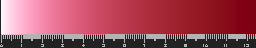Colour of colloidal metal solution in transmission: Miecolor1.for,   Sample input files: miecolin.txt, Au-n-kap.txt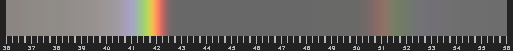Rainbows and fogbows: rainbow.for,   Sample input file: rainbdat.txt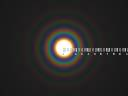Aureole: aureole.for,   Sample input file: aureolavedat.txt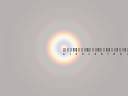Glory: glorysRGB.for,   Sample input file: glorydat.txt

Back to the overview, to the section on pigments, on rainbows or on diffraction.